# Rectangular Wire Compression Springs

## Compression Spring Design Resources – Rectangular Material

In applications where space is limited, and particularly where solid height is restricted, compression springs designed from rectangular or keystoned wire are often selected. These springs are commonly referred to as "die springs."

Springs made from rectangular wire, with the width of the rectangle perpendicular to the spring axis, store more energy in a smaller space than equivalent round wire springs. Even though stress distribution around the rectangular cross section is not as uniform as the round wire section, the energy storage capacity is higher because more material can be incorporated into the allocated space. Rectangular wire is more costly than round wire, but less costly than keystoned wire. Keystoned wire is processed specially so that deformation during spring winding or coiling causes the cross section to become approximately rectangular. Distortion of the cross section can be estimated from the equation presented below. However, distortion depends upon the manufacturing technique employed, and this equation is, at best, an approximation. Axial dimensional change of the wire must always be considered when calculating solid heights of rectangular springs.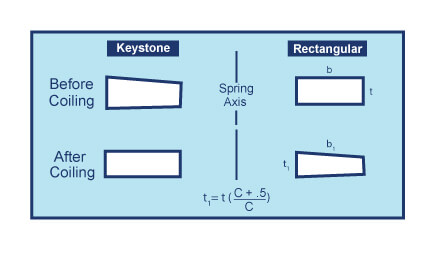The rate for a compression spring made from rectangular wire is expressed as follows: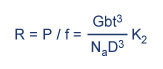Since the wire is loaded in torsion, the rate is the same whether the wire is wound on flat or on edge. Values for the constant K2 are shown in the Constants for Rectangular Wire in Torsion chart below.

Stress is expressed as: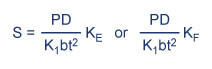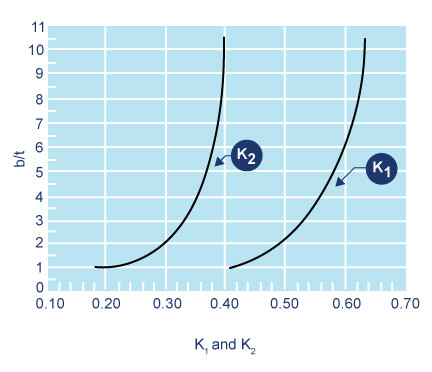Values for K1 and K2 are shown above, while values for the stress correction factor for springs wound on the flat (KF) and springs wound on edge (KE) are shown in below. When rectangular wire is produced by rolling round wire or if the cross section of the wire deviates significantly from a rectangle, additional correction factors are required. Whenever a round wire cannot be used because the solid height exceeds specifications, it is possible to try a rectangular wire coiled on edge where:

T = 2d / 1 + b / t

and d is equal to the wire diameter for the equivalent round wire spring. A typical value for a width to thickness ratio of two may be assumed in the initial design calculations.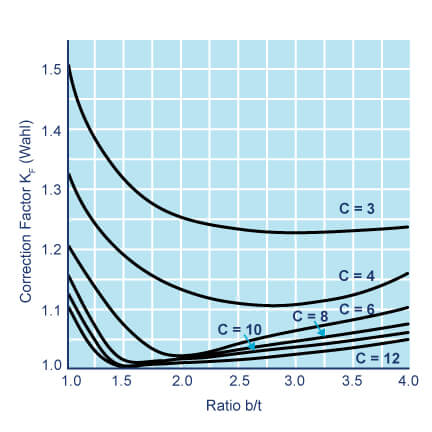Content Copyright Spring Manufacturers Institute, Inc.

This information is attributed to, and provided courtesy of, the Spring Manufacturers Institute, Inc. (SMI). Newcomb Spring and SMI provide this as advisory information only, and disclaim any and all liability of any kind for the use, application or adaption of material published on this web site.